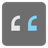End Zeros
Elementary
English JA PL RU

Try to find out how many zeros a given number has at the end.

Input: A positive Int

Output: An Int.

Example:

```end_zeros(0) == 1
end_zeros(1) == 0
end_zeros(10) == 1
end_zeros(101) == 0
```